Adverbs of Time Worksheets, ESL Activities and Games

Points of Time Questionnaire

ESL Adverbs of Time Activity - Reading, Writing, Listening and Speaking - Elementary (A1-A2) - 30 minutes

In this adverbs of time activity, students conduct a questionnaire to help them learn and practice adverbs expressing definite points of time. Students begin by writing adverbs of time from a box on a timeline. Next, students read a schedule and answers to questions. Students then write questions using adverbs of time that match the answers. After that, students answer questions based on their own schedule. Finally, students ask the questions to a partner and write down their answers.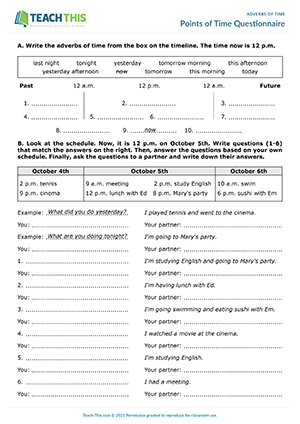Time to Learn

ESL Definite Adverbs of Time Worksheet - Reading and Writing Exercises - Pre-intermediate (A2) - 30 minutes

In this free adverbs of time worksheet, students learn and practice definite time adverbs related to points of time. To begin, students categorize six definite adverbs of time. Next, students underline the correct adverb of time in each sentence shown. After that, students rewrite sentences, correcting the position of the adverbs of time. Students then look at someone's weekly schedule and answer questions about it using time adverbs. Finally, students discuss questions with a partner using the adverbs of time from the worksheet.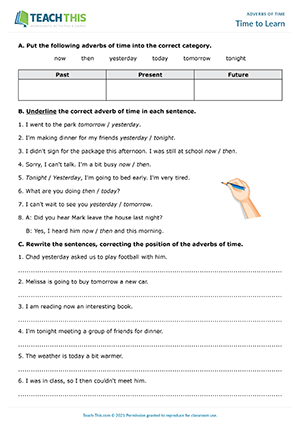Interactive Version - Here is a free time adverbs interactive worksheet to help students learn and practice definite adverbs that relate to points of time.

Definite Frequency

ESL Adverbs of Definite Frequency Worksheet - Writing and Speaking Activity - Intermediate (B1) - 30 minutes

Here is an adverbs of definite frequency worksheet for intermediate students. Students start by matching adverbs of definite frequency with definitions. Next, students read sentences about when events happen and write sentences using definite frequency adverbs to show how often each event occurs. Students then rewrite sentences, changing adjectives of frequency into adverbs of frequency. Finally, students complete sentences containing definite frequency adverbs with their own information. The students then ask a partner what they wrote and write down their answers.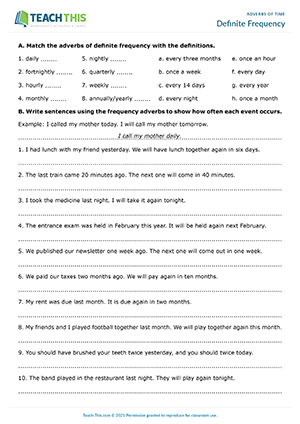Interactive Version - In this adverbs of definite frequency interactive worksheet, students complete a variety of exercises to learn definite frequency adverbs.

Relationships in Time

ESL Time Adverbs: Relationships in Time Worksheet - Reading and Writing Exercises - Intermediate (B1) - 25 minutes

This time adverbs worksheet can be used to introduce students to indefinite adverbs that demonstrate relationships in time. First, students underline the correct adverbs of time in a set of sentences. Next, students match time adverbs to their definitions. Students then use their own ideas to complete sentences with true information about themselves. After that, students do a gap-fill exercise where they complete a conversation with the adverbs of time from Exercise B. In the last exercise, students use time adverbs to answer comprehension questions about the conversation.Interactive Version - Here is a time adverbs interactive worksheet to help teach students indefinite adverbs that show relationships in time.

Time for a Review

ESL Indefinite Adverbs of Time Worksheet - Reading and Writing Exercises - Intermediate (B1) - 30 minutes

In this adverbs of time worksheet, students review indefinite time adverbs that demonstrate relationships in time. To start, students unscramble letters to make adverbs of time found in the reading. Next, students read the text and complete definitions with the correct adverbs of time. Students then read the text again and find a synonym for each time adverb shown. After that, students use the time adverbs from the previous exercises to complete sentences. Finally, students use the prompts provided to make sentences using adverbs of time.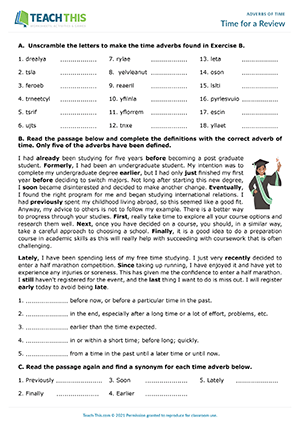Interactive Version - In this adverbs of time interactive worksheet, students work through a range of exercises to practice indefinite time adverbs that indicate relationships in time.

What Happened When?

ESL Adverbs of Time Game - Reading, Ordering and Writing Activity - Intermediate (B1) - 30 minutes

In this adverbs of time game, students race to put words in the correct order to make sentences containing adverbs of indefinite time. The first team to show and then read out each sentence in the correct order wins three points. Teams that show or read out a wrong answer lose one point. This continues until all the sentences have been completed. When the students have finished, they match the sentences with times. The first team to do this scores an additional five points. The team with the highest score at the end of the game wins.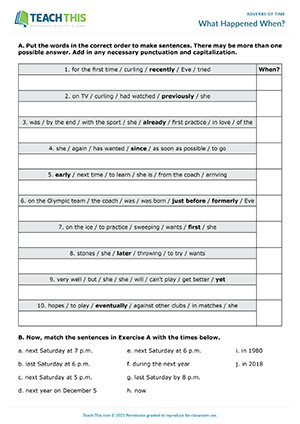0
0
0
s2sdefault